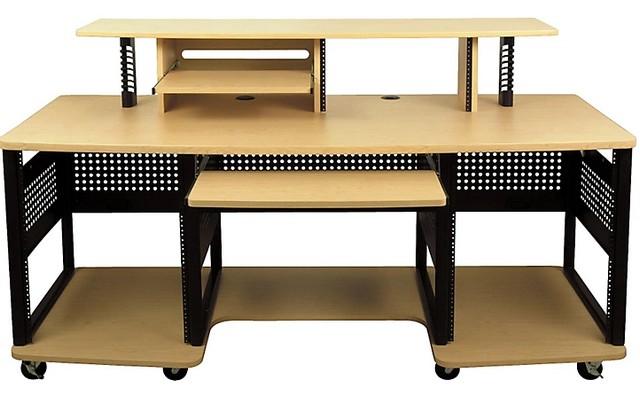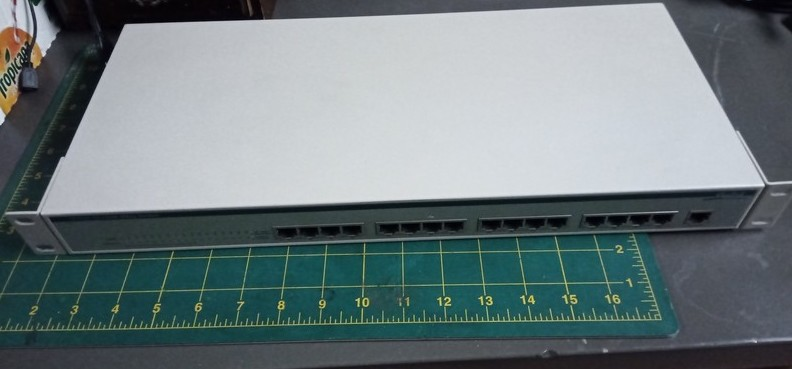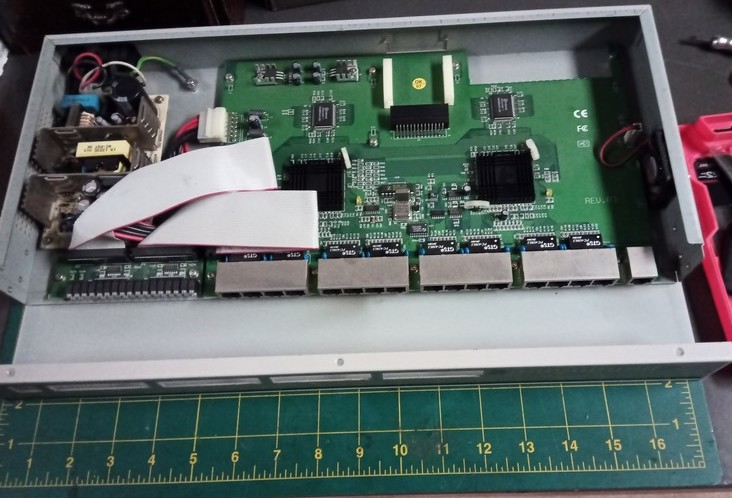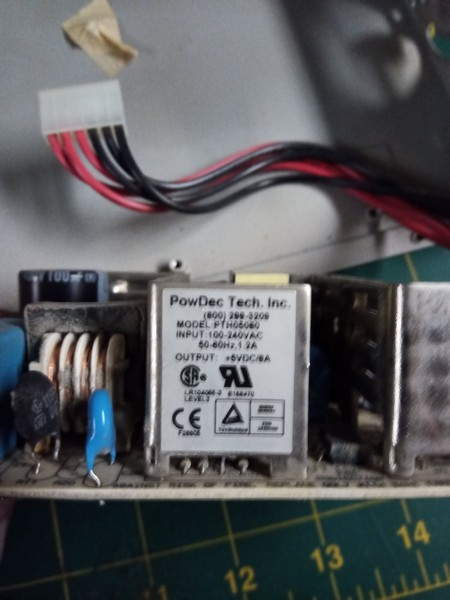```
[=x-=x=-=x=-=x=-=x=-=x=-=x=-=x=-=x=-=x=-=x=-=x=-=x=-=x=-=x=-=x=-=x=-]
[<<>><<>><<>><<>><<>><<>><<>><<>><<>><<>><<>><<>><<>><<>><<>><<>><<>]
[=x-=x=-=x=-=x=-=x=-=x=-=x=-=x=-=x=-=x=-=x=-=x=-=x=-=x=-=x=-=x=-=x=-]

Stardate: 20220118.1646
Location: xiled rumination concentrator
Input Device: xrc console
Audio: aNONradio - The Resistance with DJ bronzie94
Visual: xrc interior, interior LED colers fading, cycling.
Emotional State: alert, with m0ar coffee!

[=x-=x=-=x=-=x=-=x=-=x=-=x=-=x=-=x=-=x=-=x=-=x=-=x=-=x=-=x=-=x=-=x=-]

Continuing where I left off from my last phost 

The desk that I found at Guitar Center that was able to house my
rackmounted equipment was the Studio RTA Producer Station. I
bought the maple version.  Music studio furniture is not cheap, but I
found the price of this one to be reasonable for what I was prepared
to spend on a computer desk.  The desk has rack space on top and to
the left and the right sides of the desk.  I have disassembled and
reassembled the desk for 3 moves and it is still in great shape.  It
is still my main battlestation in the lab.Fast-forward to now.  I have aging rackmounted equipment in the lab,
some of which is active, some powered on occasionally, some filling
in rack space, and some piled up next to my desk.  I have re-gifted
some rackmounted devices previously and have kept the small pile for
parts or to repurpose.

For some time I have been contemplating repurposing one of the
4U cases to house a bunch of single board computers, but it seemed
a bit much for what I would need so I put this idea off to the side
until recently, when my year-end backups were being completed and I
needed to rebuild my NAS.  Since I had some hardware and some time,
I decided to revisit the idea and build something.  I ended up
repurposing a 1U 16-port 10/100 switch to house 2 Raspberry Pi
NAS servers.Pic: Old 1U 16-port 10/100 NWay Switch (pre-mod)

The 1U switch was in the pile next to my desk and was no longer
being used on my network.  It had noisy fan problems and was used
just for testing.  I decided to use this for the NAS.

I started opening up the case and removing the internals.  I
took out the power supply and decided to read the specifications on
the label.  Output: +5VDC @ 8A.  The Raspberry Pis run off of
5 volts DC, so I decided to repurpose the power supply as well.
Originally, I was just going to use the case and figure out
something for the power, but didn't have to, so I cleaned up the
power supply and mounted it back inside the case.Pic: Switch internalsPic: Power supply label

OK, that's it for now.  I have other matters to attend to.

 gopher://sdf.org/1/users/xiled/phlog/2022/20220117_1u_pi_nas_1of2
 https://www.guitarcenter.com/Studio-RTA/Producer-Station.gc
 Pic: Old 1U 16-port 10/100 NWay Switch (pre-mod)
 Pic: Switch internals
 Pic: Power supply label

20220120_1u_pi_nas_3of3
- 1U Raspberry Pi NAS (part 3of3)

[=x-=x=-=x=-=x=-=x=-=x=-=x=-=x=-=x=-=x=-=x=-=x=-=x=-=x=-=x=-=x=-=x=-]

```
.:[ HOME ]:.